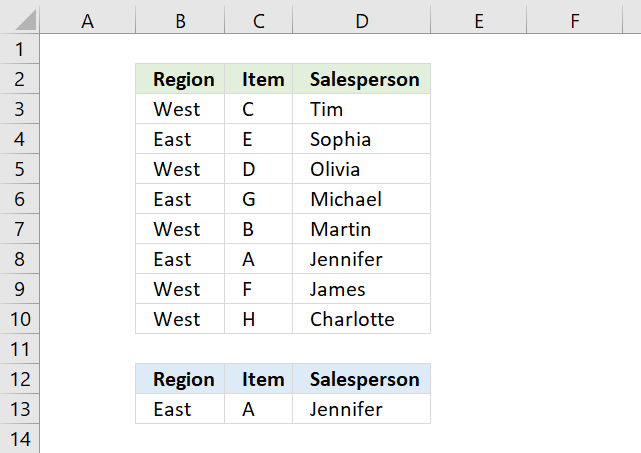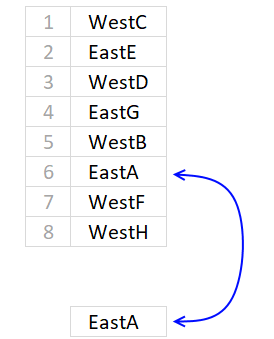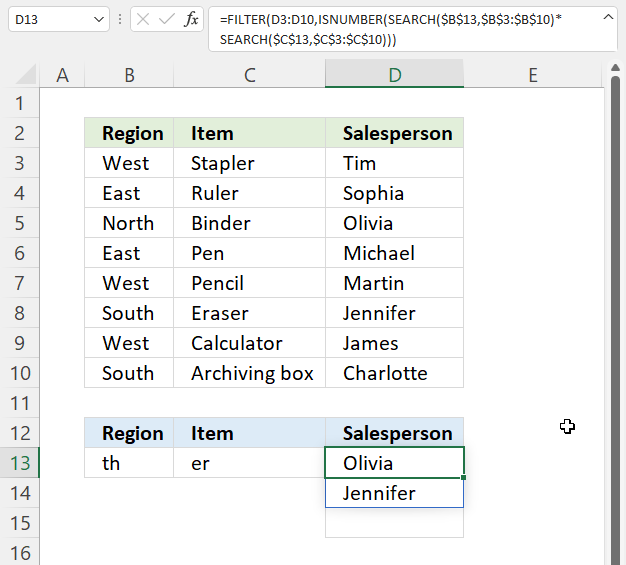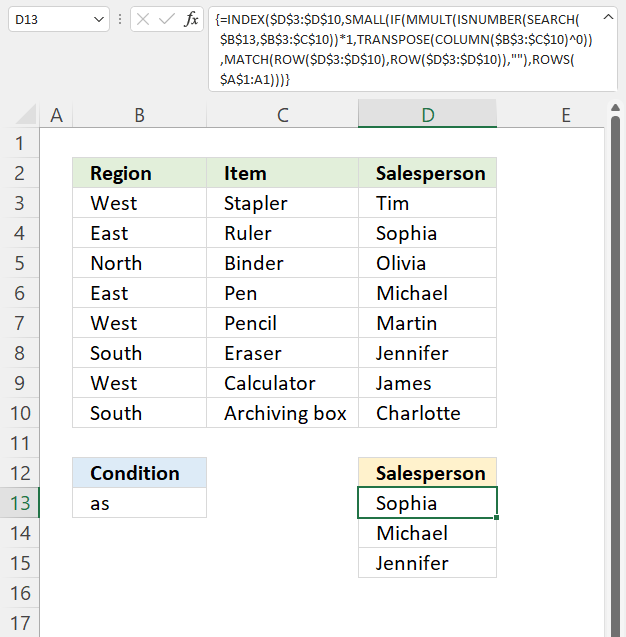Author: Oscar Cronquist Article last updated on November 22, 2021This article demonstrates formulas that let you perform lookups using two or more conditions. The image above shows two conditions in cells B13:C13, the result is in cell D13.

The formula in cell D13 returns the first match where both cells meet the conditions on the same row.

## 1. INDEX MATCH multiple criteriaThe formula demonstrated in cell D13 is a regular formula, most people prefer a regular formula over an array formula if possible, see the above picture.

=INDEX(D3:D10,MATCH(B13&C13, INDEX(B3:B10&C3:C10, ), 0))

The formula uses two conditions, one is specified in cell B13 and the other one in C13. It looks for the first condition in cell range B3 to B10 and the second condition in C3 to C10 if both conditions are met on the same row the corresponding name from cell range D3:D10 is returned.

Note, the formula returns only the first value from a row where both conditions are met. You need another formula to return values from all matching rows.

### 1.1 Explaining formula in cell D13

#### Step 1 - Concatenate lookup values

The ampersand character concatenates both values you want to look for.

B13&C13

becomes

"East"&"A"

and returns "EastA".

#### Step 2 - Concatenate lookup columns

Then it concatenates the two cell ranges also using the ampersand character, the INDEX function makes it a regular formula.

B3:B10&C3:C10

becomes

{"West"; "East"; "West"; "East"; "West"; "East"; "West"; "West"}&{"C"; "E"; "D"; "G"; "B"; "A"; "F"; "H"}

and returns

{"WestC"; "EastE"; "WestD"; "EastG"; "WestB"; "EastA"; "WestF"; "WestH"}.

#### Step 3 - Make the array formula a regular formula

The INDEX function lets you convert some array formulas to regular formulas, this is one of them.

INDEX(B3:B10&C3:C10,)The MATCH function then returns the relative position of the combined values, see picture above.

MATCH(B13&C13,INDEX(B3:B10&C3:C10,),0)

becomes

MATCH("EastA", {"WestC"; "EastE"; "WestD"; "EastG"; "WestB"; "EastA"; "WestF"; "WestH"}, 0)

and returns 6. The value is in the 6th position in the array.

#### Step 4 - Return value from the same row

INDEX(D3:D10, MATCH(B13&C13, INDEX(B3:B10&C3:C10, ), 0))

becomes

INDEX(D3:D10, 6)

becomes

INDEX({"Tim"; "Sophia"; "Olivia"; "Michael"; "Martin"; "Jennifer"; "James"; "Charlotte"}, 6)

and returns Jennifer in cell D13.

### 1.2 Array formula alternative

If you don't mind array formulas, the only advantage is that it is somewhat smaller, use this formula:

=INDEX(D3:D10, MATCH(B13&C13, B3:B10&C3:C10, 0))

To enter an array formula press and hold CTRL + SHIFT simultaneously, then press Enter once. Release all keys.

The formula bar now shows the formula enclosed with curly brackets telling you that you entered the formula successfully. Don't enter the curly brackets yourself.

## 2. INDEX MATCH - partial text multiple conditions Excel 365The formula in cell D13 checks if a cell in B3:B10 contains text specified in cell B13 and on the same row, if the corresponding cell in C3:C10 contains the specified text in C13.

The formula returns a value from D3:D10 if both cells are on the same row and contain the given text strings.

Excel 365 formula in cell D13:

=FILTER(D3:D10,ISNUMBER(SEARCH(\$B\$13,\$B\$3:\$B\$10)*SEARCH(\$C\$13,\$C\$3:\$C\$10)))

This is a dynamic array formula that works only in Excel 365, it is entered as a regular formula, however, it spills values to cells below if needed.

For previous Excel versions, see this article: Lookup with multiple criteria and return multiple search results It uses INDEX MATCH to get values based on multiple partial conditions.

### 2.1 Explaining formula in cell D13

#### Step 1 - Search for partial text in cell range B3:B10

The SEARCH function returns the number of the character at which a specific character or text string is found reading left to right (not case-sensitive)

SEARCH(find_text,within_text, [start_num])

This formula is copied to cells below in order to get all matching values. We need to use absolute references to lock cell ranges to prevent cell ranges from changing as we copy the cell and paste to cells below.

SEARCH(\$B\$13,\$B\$3:\$B\$10)

becomes

SEARCH("th",{"West"; "East"; "North"; "East"; "West"; "South"; "West"; "South"})

and returns

{#VALUE!; #VALUE!; 4; #VALUE!; #VALUE!; 4; #VALUE!; 4}.

Note that the SEARCH function returns a error value if the string is not found in a cell value.

#### Step 2 - Search for second partial text condition in cell range C3:C10

SEARCH(\$C\$13, \$C\$3:\$C\$10)

becomes

SEARCH("er",{"Stapler"; "Ruler"; "Binder"; "Pen"; "Pencil"; "Eraser"; "Calculator"; "Archiving box"})

and returns

{6; 4; 5; #VALUE!; #VALUE!; 1; #VALUE!; #VALUE!}.

#### Step 3 - Multiply arrays

We will mutiply both arrays to perform AND logic by using the asterisk character.

SEARCH(\$B\$13, \$B\$3:\$B\$10)*SEARCH(\$C\$13, \$C\$3:\$C\$10))

becomes

{#VALUE!; #VALUE!; 4; #VALUE!; #VALUE!; 4; #VALUE!; 4} * {6; 4; 5; #VALUE!; #VALUE!; 1; #VALUE!; #VALUE!}

and returns

{#VALUE!; #VALUE!; 20; #VALUE!; #VALUE!; 4; #VALUE!; #VALUE!}.

A number multipled with an error value returns a error value, however, a number multipled with a number returns a number.

#### Step 4 - Check if a value in the array is a number

The ISNUMBER function returns TRUE or FALSE based on the contents of the argument.

ISNUMBER(SEARCH(\$B\$13, \$B\$3:\$B\$10)*SEARCH(\$C\$13, \$C\$3:\$C\$10))

becomes

ISNUMBER({#VALUE!; #VALUE!; 20; #VALUE!; #VALUE!; 4; #VALUE!; #VALUE!})

and returns

{FALSE; FALSE; TRUE; FALSE; FALSE; TRUE; FALSE; FALSE}.

#### Step 4 - Extract values based on logical array

The FILTER function returns values/rows based on a condition or criteria, it is only availabe to Excel 365 subscribers.

FILTER(D3:D10, ISNUMBER(SEARCH(\$B\$13, \$B\$3:\$B\$10)*SEARCH(\$C\$13, \$C\$3:\$C\$10)))

becomes

FILTER(D3:D10, {FALSE; FALSE; TRUE; FALSE; FALSE; TRUE; FALSE; FALSE})

becomes

FILTER({"Tim"; "Sophia"; "Olivia"; "Michael"; "Martin"; "Jennifer"; "James"; "Charlotte"}, {FALSE; FALSE; TRUE; FALSE; FALSE; TRUE; FALSE; FALSE})

and returns {"Olivia"; "Jennifer"}.

## 3. INDEX MATCH - partial match multiple columnsThe array formula in cell D13 extracts values from column D if the corresponding value in cell range B3:C3 contains the specified string in cell B13.

The condition in cell B13 is found in cells B4, B6, and C8. The corresponding values in column D are in D4, D6, and D8.

Array formula in cell D13:

=INDEX(\$D\$3:\$D\$10, SMALL(IF(MMULT(ISNUMBER(SEARCH(\$B\$13, \$B\$3:\$C\$10))*1, TRANSPOSE(COLUMN(\$B\$3:\$C\$10)^0)), MATCH(ROW(\$D\$3:\$D\$10), ROW(\$D\$3:\$D\$10)), ""), ROWS(\$A\$1:A1)))

### 3.1 Explaining formula in cell D13

#### Step 1 - Search cell range \$B\$3:\$C\$10 for string

The SEARCH function returns the number of the character at which a specific character or text string is found reading left to right (not case-sensitive)

SEARCH(find_text,within_text, [start_num])

SEARCH(\$B\$13, \$B\$3:\$C\$10)

becomes

SEARCH("as",{"West","Stapler"; "East","Ruler"; "North","Binder"; "East","Pen"; "West","Pencil"; "South","Eraser"; "West","Calculator"; "South","Archiving box"})

and returns

{#VALUE!,#VALUE!; 2,#VALUE!; #VALUE!,#VALUE!; 2,#VALUE!; #VALUE!,#VALUE!; #VALUE!,3; #VALUE!,#VALUE!; #VALUE!,#VALUE!}

#### Step 2 - Identify numbers in array

The ISNUMBER function returns TRUE or FALSE based on the contents of the argument.

ISNUMBER(SEARCH(\$B\$13, \$B\$3:\$C\$10))*1

becomes

ISNUMBER({#VALUE!,#VALUE!; 2,#VALUE!; #VALUE!,#VALUE!; 2,#VALUE!; #VALUE!,#VALUE!; #VALUE!,3; #VALUE!,#VALUE!; #VALUE!,#VALUE!})*1

becomes

{FALSE,FALSE; TRUE,FALSE; FALSE,FALSE; TRUE,FALSE; FALSE,FALSE; FALSE,TRUE; FALSE,FALSE; FALSE,FALSE}*1

The MMULT function can't calculate boolean values like TRUE and FALSE, we need to convert them to their numerical equivalents. TRUE - 1 , and FALSE - 0 (zero)

{FALSE,FALSE; TRUE,FALSE; FALSE,FALSE; TRUE,FALSE; FALSE,FALSE; FALSE,TRUE; FALSE,FALSE; FALSE,FALSE}*1

returns

{0,0; 1,0; 0,0; 1,0; 0,0; 0,1; 0,0; 0,0}.

#### Step 3 - Create an array containing 1

TRANSPOSE(COLUMN(\$B\$3:\$C\$10)^0)

The COLUMN function returns row numbers based on a cell range.

COLUMN(\$B\$3:\$C\$10)

returns {2,3}.

COLUMN(\$B\$3:\$C\$10)^0

returns {1,1}.

TRANSPOSE(COLUMN(\$B\$3:\$C\$10)^0)

becomes

TRANSPOSE({1, 1})

and returns {1; 1}.

#### Step 4 - Add values column-wise

The MMULT function calculates the matrix product of two arrays, an array as the same number of rows as array1 and columns as array2.

MMULT(array1array2)

MMULT(ISNUMBER(SEARCH(\$B\$13, \$B\$3:\$C\$10))*1, TRANSPOSE(COLUMN(\$B\$3:\$C\$10)^0))

becomes

MMULT({0,0; 1,0; 0,0; 1,0; 0,0; 0,1; 0,0; 0,0}, {1; 1})

and returns {0; 1; 0; 1; 0; 1; 0; 0}.

#### Step 5 - Create an array from 1 to n

The ROW function lets you create numbers representing the rows based on a cell range.

MATCH(ROW(\$D\$3:\$D\$10),ROW(\$D\$3:\$D\$10))

becomes

MATCH({3; 4; 5; 6; 7; 8; 9; 10}, {3; 4; 5; 6; 7; 8; 9; 10})

The MATCH function finds the relative position of a given string in an array or cell range. This will create an array from 1 to n where n is the number of rows in cell range \$D\$3:\$D\$10.

MATCH({3; 4; 5; 6; 7; 8; 9; 10}, {3; 4; 5; 6; 7; 8; 9; 10})

and returns {1; 2; 3; 4; 5; 6; 7; 8}. There are eight rows in \$D\$3:\$D\$10.

#### Step 6 - Replace 1 with corresponding row number

The IF function returns one value if the logical test is TRUE and another value if the logical test is FALSE.

IF(logical_test, [value_if_true], [value_if_false])

IF(MMULT(ISNUMBER(SEARCH(\$B\$13, \$B\$3:\$C\$10))*1, TRANSPOSE(COLUMN(\$B\$3:\$C\$10)^0)), MATCH(ROW(\$D\$3:\$D\$10), ROW(\$D\$3:\$D\$10)), "")

becomes

IF({0; 1; 0; 1; 0; 1; 0; 0}, {1; 2; 3; 4; 5; 6; 7; 8}, "")

and returns {""; 2; ""; 4; ""; 6; ""; ""}.

#### Step 7 - Extract k-th smallest row number

The SMALL function returns the k-th smallest value from a group of numbers.

SMALL(array, k)

SMALL(IF(MMULT(ISNUMBER(SEARCH(\$B\$13, \$B\$3:\$C\$10))*1, TRANSPOSE(COLUMN(\$B\$3:\$C\$10)^0)), MATCH(ROW(\$D\$3:\$D\$10), ROW(\$D\$3:\$D\$10)), ""), ROWS(\$A\$1:A1))

becomes

SMALL({""; 2; ""; 4; ""; 6; ""; ""}, ROWS(\$A\$1:A1))

becomes

SMALL({""; 2; ""; 4; ""; 6; ""; ""}, 1)

and returns 2.

#### Step 8 - Get values based on row number

The INDEX function returns a value from a cell range, you specify which value based on a row and column number.

INDEX(\$D\$3:\$D\$10, SMALL(IF(MMULT(ISNUMBER(SEARCH(\$B\$13, \$B\$3:\$C\$10))*1, TRANSPOSE(COLUMN(\$B\$3:\$C\$10)^0)), MATCH(ROW(\$D\$3:\$D\$10), ROW(\$D\$3:\$D\$10)), ""), ROWS(\$A\$1:A1)))

becomes

INDEX(\$D\$3:\$D\$10, 2)

becomes

INDEX({"Tim"; "Sophia"; "Olivia"; "Michael"; "Martin"; "Jennifer"; "James"; "Charlotte"}, 2)

and returns "Sophia".

The following formula is an Excel 365 dynamic array formula:

=FILTER(D3:D10, MMULT(ISNUMBER(SEARCH(B13, B3:C10))*1, TRANSPOSE(COLUMN(B3:C10)^0)))

Absolute cell references are not required, the formula returns an array that spills values to cells below automatically.

## 4. Get Excel file

### Get the Excel fileINDEX-MATCH-multiple-conditionsv2.xlsx